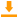# Minimum

 Block Group: Statistical Functions Icon:The Minimum block returns the smallest value in a list of arguments.

#### Note

If none of the input n values is a number, the block outputs zero.

For information on using dataflow blocks, see Dataflow.

For accepted and excluded arguments of Statistical Functions blocks, see Statistical Functions.

## Input/Output Property

The following property of the Minimum block can take input and give output.

• input n (number)

input n specifies one of the input values for the statistical function.

## Output Property

The following property of the Minimum block can give output but cannot take input.

• output (number)

output returns the smallest of the input values.

## Example

The following image shows an example of the Minimum block.